## 【LeetCode热题100】打卡第21天：最小路径和爬楼梯

article2023/10/2 20:21:17/

### 文章目录

• 【LeetCode热题100】打卡第21天：最小路径和&爬楼梯
• ⛅前言
• 最小路径和
• 🔒题目
• 爬楼梯
• 🔒题目
• 🔑题解

# 【LeetCode热题100】打卡第21天：最小路径和&爬楼梯

## ⛅前言

LeetCode热题100专栏🚀：LeetCode热题100

Gitee地址📁：知识汲取者 (aghp) - Gitee.com

PS：作者水平有限，如有错误或描述不当的地方，恳请及时告诉作者，作者将不胜感激

# 最小路径和

## 🔒题目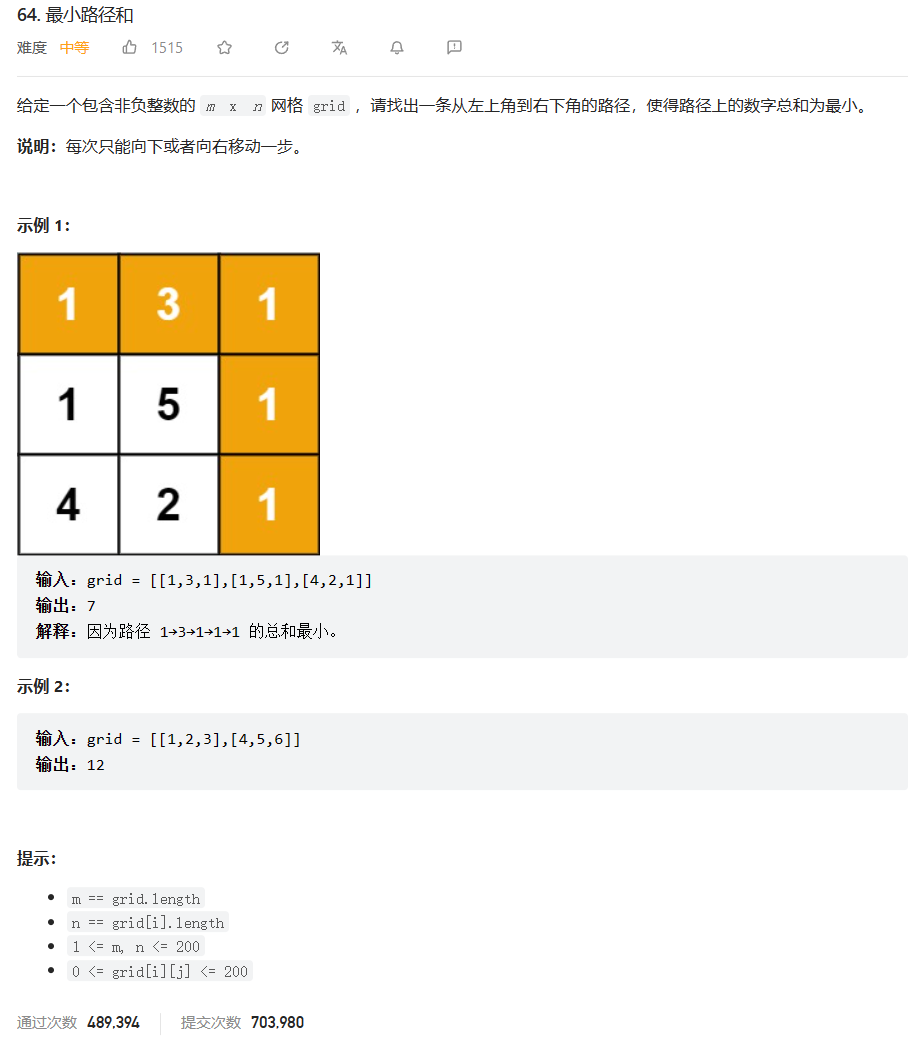• 解法一：动态规划

题目分析：①辨别题目的类型。通过阅读并辨别（这个需要学习并做过动态规划这类题型的经验，本体比较好辩别），我们可以发现这是一个典型的动态规划问题，可以使用一个db数组缓存当前节点的最短距离，然后下一个节点就可以复用，而不需要重新去计算。②思考对应的解法。既然我们已经知道，这是一个动态规划问题，剩下的就是推导出转移方程。当前节点的状态，有两个来源，要么从上边来，要么从左边来，因为是最小路径，所以我们使用 M a t h . m i n ( d b [ i − 1 ] [ j ] , d b [ i ] [ j − 1 ] ) Math.min(db[i - 1][j], db[i][j - 1]) 判断当前节点的来源，其次还需要加上当前节点的距离，最终是 d b [ i ] [ j ] = g r i d [ i − 1 ] [ j − 1 ] + M a t h . m i n ( d b [ i − 1 ] [ j ] , d b [ i ] [ j − 1 ] ) db[i][j] = grid[i - 1][j - 1] + Math.min(db[i - 1][j], db[i][j - 1]) 。③完善逻辑。通过②可以得到了通用节点的转移方程，但是对于 i==1j==1这两种情况，我们需要单独考虑，因为他们有边界，上边和左边没有元素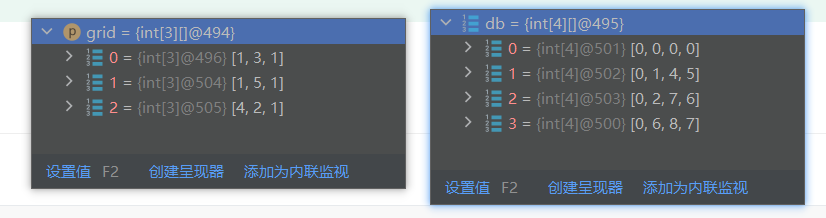/**
* @author ghp
* @title 不同路径
*/
class Solution {
public int minPathSum(int[][] grid) {
int m = grid.length;
int n = grid.length;
int[][] db = new int[m + 1][n + 1];
for (int i = 1; i <= m; i++) {
for (int j = 1; j <= n; j++) {
if (i == 1 || j == 1) {
db[i][j] = grid[i - 1][j - 1] + Math.max(db[i - 1][j], db[i][j - 1]);
} else {
db[i][j] = grid[i - 1][j - 1] + Math.min(db[i - 1][j], db[i][j - 1]);
}
}
}
return db[m][n];
}
}


复杂度分析

时间复杂度： O ( m ∗ n ) O(m*n)

空间复杂度： O ( m ∗ n ) O(m*n)

• 解法二：DFS+记忆搜索

首先最短路径问题，肯定是可以使用DFS和BFS的，但是直接暴力DFS或BFS是肯定行不通的，这里需要使用记忆搜搜。所谓的记忆搜索很简单，就是在搜索的过程中缓存当前搜索的结果，这样就能减少很多重复性的搜索，从而大大提高搜索效率

import java.util.Arrays;

/**
* @author ghp
* @title 不同路径
*/
class Solution {
public int minPathSum(int[][] grid) {
int m = grid.length;
int n = grid.length;
int[][] path = new int[m][n];
// 初始化path数组
for (int i = 0; i < path.length; i++) {
Arrays.fill(path[i], -1);
}
path[m - 1][n - 1] = grid[m - 1][n - 1];
return dfs(grid, path, 0, 0);
}

/**
* 深度搜索
*
* @param grid 待搜索的图
* @param path 用于记录当前每次搜索的最短路径
* @param r    行
* @param c    列
* @return （0,0）到 (m-1,n-1) 的最短路径
*/
private int dfs(int[][] grid, int[][] path, int r, int c) {
if (r >= grid.length || c >= grid.length) {
// 越界
return Integer.MAX_VALUE;
}
if (path[r][c] != -1) {
// 该点已经走过，直接返回当前点到达终点的最短路径
return path[r][c];
}
// 往下
int down = dfs(grid, path, r + 1, c);
// 往右
int right = dfs(grid, path, r, c + 1);
// 记录当前节点到达终点的最短路径（核心步骤）
path[r][c] = grid[r][c] + Math.min(right, down);
return path[r][c];
}
}


复杂度分析

时间复杂度： O ( m ∗ n ) O(m*n)

空间复杂度： O ( m ∗ n ) O(m*n)

• 解法三：BFS+记忆搜索

import java.util.Arrays;
import java.util.Queue;

/**
* @author ghp
* @title 不同路径
*/
class Solution {
public int minPathSum(int[][] grid) {
int[][] path = new int[grid.length][grid.length];
// 初始化记忆数组
for (int[] row : path) {
Arrays.fill(row, -1);
}
path = grid;
return bfs(path, grid);
}

private static int bfs(int[][] path, int[][] grid) {
int m = grid.length;
int n = grid.length;
queue.offer(new int[]{0, 0}); // 出发点
// 方向向量，向左，向下
int[][] dirs = {{0, 1}, {1, 0}};
// 开始进行广度搜索
while (!queue.isEmpty()) {
int[] curr = queue.poll();
int x = curr;
int y = curr;
// 遍历向左和向下的点
for (int[] dir : dirs) {
int dx = x + dir;
int dy = y + dir;
if (dx >= 0 && dx < m && dy >= 0 && dy < n) {
// 当前没有发生越界
if (path[dx][dy] == -1 || path[dx][dy] > path[x][y] + grid[dx][dy]) {
// 当前点没有被遍历 或 当前路径长度比之前路径更短，都需要更新最短路径
path[dx][dy] = path[x][y] + grid[dx][dy];
// 将当前所在坐标加入到队列中，方便遍历下一个节点
queue.offer(new int[]{dx, dy});
}
}
}
}
return path[m - 1][n - 1];
}
}


复杂度分析

时间复杂度： O ( m ∗ n ) O(m*n)

空间复杂度： O ( m ∗ n ) O(m*n)

PS：通过提交检测，可以发现虽然三者的时间复杂度和空间复杂度都是一样的，但是时间上和空间上最优的是动态规划，其次是DFS，最好才是BFS。实现起来最复杂的是BFS，其次是DFS，最后才是动态规划，所以综上所述，本题的最优解是动态规划，LeetCode官方也只提供了动态规划的题解(●ˇ∀ˇ●)

# 爬楼梯

## 🔒题目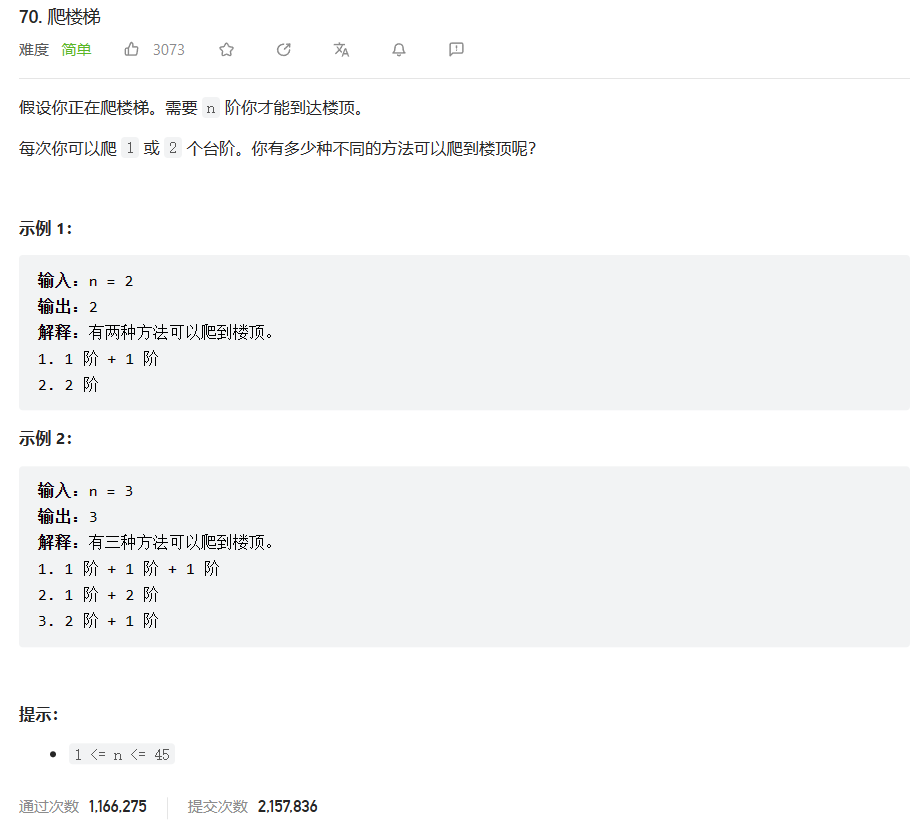## 🔑题解

• 解法一：暴力DFS（示例数据为44时时间超限）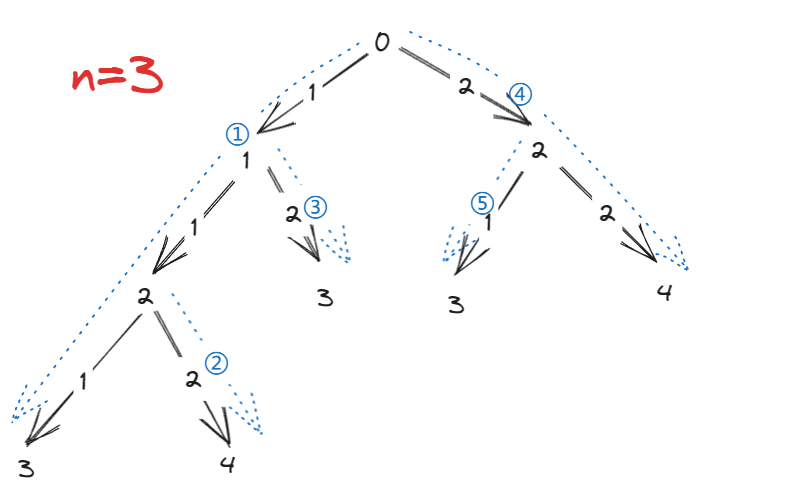/**
* @author ghp
* @title 爬楼梯
*/
class Solution {
private int count = 0;
public int climbStairs(int n) {
dfs(n, 0);
return count;
}

private void dfs(int n, int path) {
if (path == n){
count++;
return;
}
if (path > n){
return;
}
for (int i = 1; i <= 2; i++) {
dfs(n, path+i);
}
}
}


复杂度分析：

• 时间复杂度： O ( 2 n ) O(2^n)
• 空间复杂度： O ( n ) O(n)

代码优化：DFS+记忆搜索

可以使用记忆化搜索来优化这段代码，避免重复计算。具体实现如下：

1. 创建一个记忆数组 memo，将每个位置初始化为 -1，表示没有计算过。
2. 在 dfs 函数中，首先判断 memo 数组中当前状态是否计算过，若已计算过则直接返回对应的值。
3. 如果 memo 数组中当前状态未计算过，则进行正常的搜索，并在结束搜索后将结果保存到 memo 数组中。
4. 最后返回结果即可。

主要思想，因为DFS搜索的结果是一颗二叉树，二叉树具有对称性，当我们在左边搜索过结果，右侧搜索到同样的结果时，可以直接不需要计算，直接使用之前的计算结果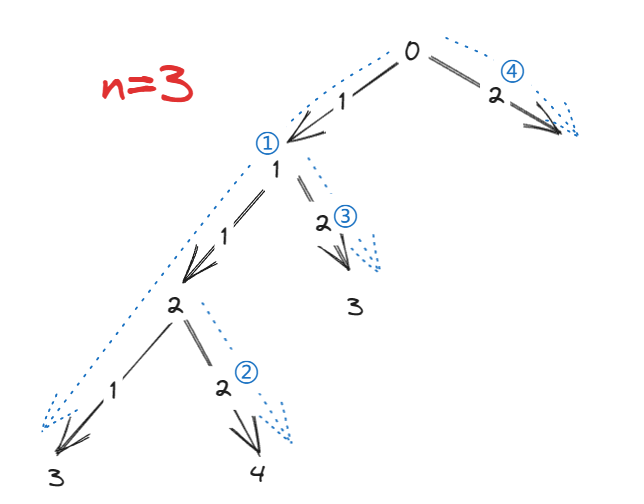import java.util.Arrays;

/**
* @author ghp
* @title 爬楼梯
*/
class Solution {
private int count = 0; // 记录可达的路径条数
private int[] memo; // 记录当前节点可达的路径条数

public int climbStairs(int n) {
memo = new int[n + 1];
Arrays.fill(memo, -1);
dfs(n, 0);
return count;
}

private void dfs(int n, int path) {
if (path == n) {
// 当前路径符合，路径条数+1
count++;
return;
}
if (path > n) {
// 已经到底了，无法继续往下遍历
return;
}
if (memo[path] != -1) {
// 当前节点被遍历过，则不需要继续往下遍历
count += memo[path];
return;
}
// 遍历当前节点下的左右子树
for (int i = 1; i <= 2; i++) {
dfs(n, path + i);
}
// 将当前节点可达的路径总数保存到 memo 数组中
memo[path] = count;
}
}


复杂度分析：

时间复杂度和空间复杂度和之前是一样的，通过存储每次搜索的状态，我们可以减少很多重复的搜索

• 解法二：动态规划

每一个阶梯都有两个状态，要么来自上一个（走一步），要么来自上上一个（走两步），所以我们可以得到状态转移方程： f ( x ) = f ( x − 1 ) + f ( x − 2 ) f(x)=f(x-1)+f(x-2) ，通过枚举，可以发现 f ( 1 ) = 1 , f ( 2 ) = 2 , f ( 3 ) = 3 , f ( 4 ) = 5... f(1)=1,f(2)=2,f(3)=3,f(4)=5... 显然，这是一个斐波那契数列！

class Solution {
public int climbStairs(int n) {
int p = 0, q = 0, r = 1;
for (int i = 1; i <= n; ++i) {
p = q;
q = r;
r = p + q;
}
return r;
}
}


复杂度分析：

• 时间复杂度： O ( n ) O(n)
• 空间复杂度： O ( 1 ) O(1)
• 解法三：公式法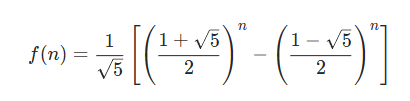### wsl安装ubuntu并设置gnome图形界面详细步骤（win11+ubuntu18）

0.前言 wsl确实是个好东西&#xff0c;不过之前配了好几次都没有成功&#xff0c;因为wsl本身确实是有bug。当时配的时候查到GitHub上的一个issue还没被修好。现在重新配一下。 我的环境是Windows11家庭版。区别于win10&#xff0c;win11安装完默认就是wsl2。 1.下载 首先打…

### 3.数据操作

SQL句子中语法格式提示&#xff1a; 1.中括号&#xff08;[]&#xff09;中的内容为可选项&#xff1b; 2.[&#xff0c;...]表示&#xff0c;前面的内容可重复&#xff1b; 3.大括号&#xff08;{}&#xff09;和竖线&#xff08;|&#xff09;表示选择项&#xff0c;在选择…

### 键盘按键事件 通过键盘上下左右按键移动界面上图标

#main.c文件 #include “keyevent.h” #include int main(int argc, char *argv[]) { QApplication a(argc, argv); KeyEvent w; w.show(); return a.exec();} #include “keyevent.h”//头文件 #ifndef KEYEVENT_H #define KEYEVENT_H #include #include #include cl…

### chatgpt赋能python：Python怎么退出程序：让你轻松掌握退出Python程序的方法

Python怎么退出程序&#xff1a;让你轻松掌握退出Python程序的方法 Python是一种功能强大、易于学习且具有广泛应用的编程语言。在Python开发中&#xff0c;经常需要退出程序&#xff0c;以便在不需要时释放内存和其他资源。那么&#xff0c;Python怎么退出程序&#xff1f;本…

### Baumer工业相机堡盟工业相机如何使用BGAPISDK的相机图像时间戳计算运行时间以及时间差（C#）

Baumer工业相机堡盟工业相机如何使用BGAPISDK的相机图像时间戳计算运行时间以及时间差&#xff08;C#&#xff09; Baumer工业相机Baumer工业相机BGAPI SDK和图像时间戳的技术背景Baumer工业相机使用BGAPISDK控制相机数据流的方式1.引用合适的类文件2.使用BGAPISDK获取时间戳的…

### CloudQuery一体化数据库SQL操作安全管控平台

&#x1f497;wei_shuo的个人主页 &#x1f4ab;wei_shuo的学习社区 &#x1f310;Hello World &#xff01; CloudQuery一体化数据库SQL操作安全管控平台 导读 CloudQuery作为业界领先的面向企业的数据库安全解决方案&#xff0c;CloudQuery致力于打造一站式安全可靠的数据操…